# Basics of Thermodynamics

12. Sep 2021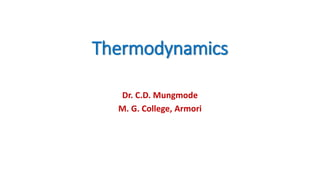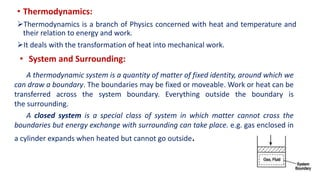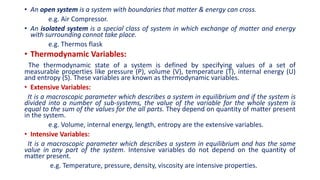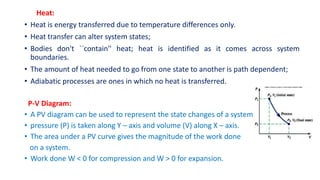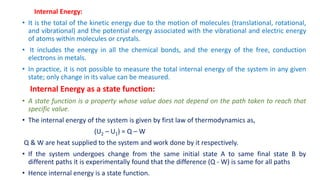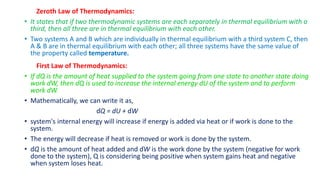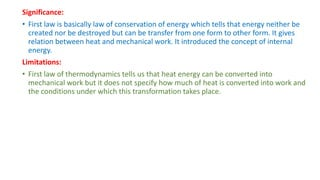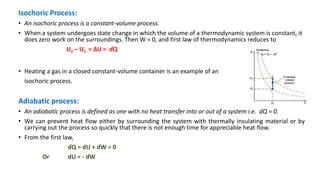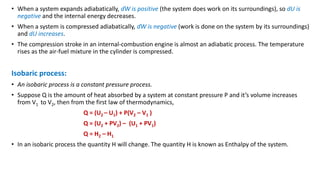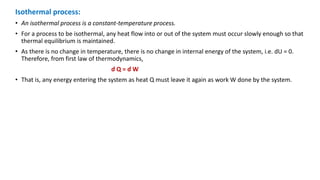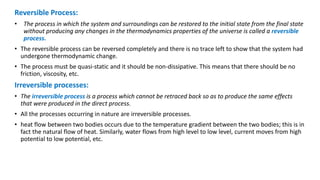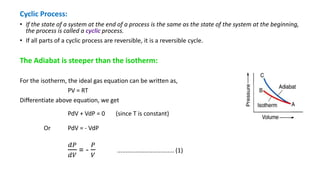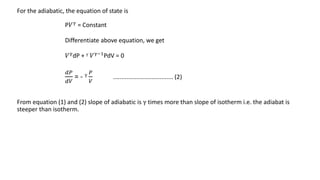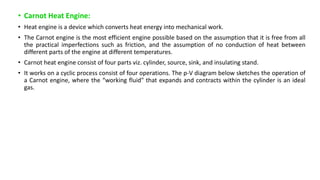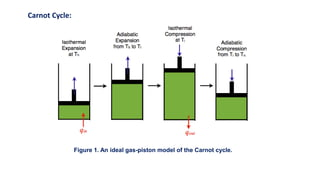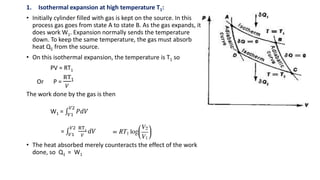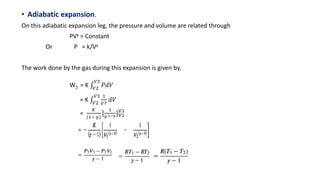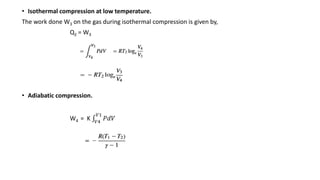1 von 18

### Basics of Thermodynamics

• 1. Thermodynamics Dr. C.D. Mungmode M. G. College, Armori
• 2. • Thermodynamics: Thermodynamics is a branch of Physics concerned with heat and temperature and their relation to energy and work. It deals with the transformation of heat into mechanical work. • System and Surrounding: A thermodynamic system is a quantity of matter of fixed identity, around which we can draw a boundary. The boundaries may be fixed or moveable. Work or heat can be transferred across the system boundary. Everything outside the boundary is the surrounding. A closed system is a special class of system in which matter cannot cross the boundaries but energy exchange with surrounding can take place. e.g. gas enclosed in a cylinder expands when heated but cannot go outside.
• 3. • An open system is a system with boundaries that matter & energy can cross. e.g. Air Compressor. • An isolated system is a special class of system in which exchange of matter and energy with surrounding cannot take place. e.g. Thermos flask • Thermodynamic Variables: The thermodynamic state of a system is defined by specifying values of a set of measurable properties like pressure (P), volume (V), temperature (T), internal energy (U) and entropy (S). These variables are known as thermodynamic variables. • Extensive Variables: It is a macroscopic parameter which describes a system in equilibrium and if the system is divided into a number of sub-systems, the value of the variable for the whole system is equal to the sum of the values for the all parts. They depend on quantity of matter present in the system. e.g. Volume, internal energy, length, entropy are the extensive variables. • Intensive Variables: It is a macroscopic parameter which describes a system in equilibrium and has the same value in any part of the system. Intensive variables do not depend on the quantity of matter present. e.g. Temperature, pressure, density, viscosity are intensive properties.
• 4. Heat: • Heat is energy transferred due to temperature differences only. • Heat transfer can alter system states; • Bodies don't ``contain'' heat; heat is identified as it comes across system boundaries. • The amount of heat needed to go from one state to another is path dependent; • Adiabatic processes are ones in which no heat is transferred. P-V Diagram: • A PV diagram can be used to represent the state changes of a system. • pressure (P) is taken along Y – axis and volume (V) along X – axis. • The area under a PV curve gives the magnitude of the work done on a system. • Work done W < 0 for compression and W > 0 for expansion.
• 5. Internal Energy: • It is the total of the kinetic energy due to the motion of molecules (translational, rotational, and vibrational) and the potential energy associated with the vibrational and electric energy of atoms within molecules or crystals. • It includes the energy in all the chemical bonds, and the energy of the free, conduction electrons in metals. • In practice, it is not possible to measure the total internal energy of the system in any given state; only change in its value can be measured. Internal Energy as a state function: • A state function is a property whose value does not depend on the path taken to reach that specific value. • The internal energy of the system is given by first law of thermodynamics as, (U2 – U1) = Q – W Q & W are heat supplied to the system and work done by it respectively. • If the system undergoes change from the same initial state A to same final state B by different paths it is experimentally found that the difference (Q - W) is same for all paths • Hence internal energy is a state function.
• 6. Zeroth Law of Thermodynamics: • It states that if two thermodynamic systems are each separately in thermal equilibrium with a third, then all three are in thermal equilibrium with each other. • Two systems A and B which are individually in thermal equilibrium with a third system C, then A & B are in thermal equilibrium with each other; all three systems have the same value of the property called temperature. First Law of Thermodynamics: • If ɗQ is the amount of heat supplied to the system going from one state to another state doing work ɗW, then ɗQ is used to increase the internal energy dU of the system and to perform work dW. • Mathematically, we can write it as, ɗQ = dU + ɗW • system's internal energy will increase if energy is added via heat or if work is done to the system. • The energy will decrease if heat is removed or work is done by the system. • ɗQ is the amount of heat added and ɗW is the work done by the system (negative for work done to the system), Q is considering being positive when system gains heat and negative when system loses heat.
• 7. Significance: • First law is basically law of conservation of energy which tells that energy neither be created nor be destroyed but can be transfer from one form to other form. It gives relation between heat and mechanical work. It introduced the concept of internal energy. Limitations: • First law of thermodynamics tells us that heat energy can be converted into mechanical work but it does not specify how much of heat is converted into work and the conditions under which this transformation takes place.
• 8. Isochoric Process: • An isochoric process is a constant-volume process. • When a system undergoes state change in which the volume of a thermodynamic system is constant, it does zero work on the surroundings. Then W = 0, and first law of thermodynamics reduces to U2 – U1 = ΔU = ɗQ • Heating a gas in a closed constant-volume container is an example of an isochoric process. Adiabatic process: • An adiabatic process is defined as one with no heat transfer into or out of a system i.e. ɗQ = 0. • We can prevent heat flow either by surrounding the system with thermally insulating material or by carrying out the process so quickly that there is not enough time for appreciable heat flow. • From the first law, ɗQ = dU + ɗW = 0 Or dU = - ɗW
• 9. • When a system expands adiabatically, ɗW is positive (the system does work on its surroundings), so dU is negative and the internal energy decreases. • When a system is compressed adiabatically, ɗW is negative (work is done on the system by its surroundings) and dU increases. • The compression stroke in an internal-combustion engine is almost an adiabatic process. The temperature rises as the air-fuel mixture in the cylinder is compressed. Isobaric process: • An isobaric process is a constant pressure process. • Suppose Q is the amount of heat absorbed by a system at constant pressure P and it’s volume increases from V1 to V2, then from the first law of thermodynamics, Q = (U2 – U1) + P(V2 – V1 ) Q = (U2 + PV2) – (U1 + PV1) Q = H2 – H1 • In an isobaric process the quantity H will change. The quantity H is known as Enthalpy of the system.
• 10. Isothermal process: • An isothermal process is a constant-temperature process. • For a process to be isothermal, any heat flow into or out of the system must occur slowly enough so that thermal equilibrium is maintained. • As there is no change in temperature, there is no change in internal energy of the system, i.e. dU = 0. Therefore, from first law of thermodynamics, ɗ Q = ɗ W • That is, any energy entering the system as heat Q must leave it again as work W done by the system.
• 11. Reversible Process: • The process in which the system and surroundings can be restored to the initial state from the final state without producing any changes in the thermodynamics properties of the universe is called a reversible process. • The reversible process can be reversed completely and there is no trace left to show that the system had undergone thermodynamic change. • The process must be quasi-static and it should be non-dissipative. This means that there should be no friction, viscosity, etc. Irreversible processes: • The irreversible process is a process which cannot be retraced back so as to produce the same effects that were produced in the direct process. • All the processes occurring in nature are irreversible processes. • heat flow between two bodies occurs due to the temperature gradient between the two bodies; this is in fact the natural flow of heat. Similarly, water flows from high level to low level, current moves from high potential to low potential, etc.
• 12. Cyclic Process: • If the state of a system at the end of a process is the same as the state of the system at the beginning, the process is called a cyclic process. • If all parts of a cyclic process are reversible, it is a reversible cycle. The Adiabat is steeper than the isotherm: For the isotherm, the ideal gas equation can be written as, PV = RT Differentiate above equation, we get PdV + VdP = 0 (since T is constant) Or PdV = - VdP 𝑑𝑃 𝑑𝑉 = - 𝑃 𝑉 .................................. (1)
• 13. For the adiabatic, the equation of state is P𝑉γ = Constant Differentiate above equation, we get 𝑉γ dP + γ 𝑉γ−1 PdV = 0 𝑑𝑃 𝑑𝑉 = - γ 𝑃 𝑉 .................................... (2) From equation (1) and (2) slope of adiabatic is γ times more than slope of isotherm i.e. the adiabat is steeper than isotherm.
• 14. • Carnot Heat Engine: • Heat engine is a device which converts heat energy into mechanical work. • The Carnot engine is the most efficient engine possible based on the assumption that it is free from all the practical imperfections such as friction, and the assumption of no conduction of heat between different parts of the engine at different temperatures. • Carnot heat engine consist of four parts viz. cylinder, source, sink, and insulating stand. • It works on a cyclic process consist of four operations. The p-V diagram below sketches the operation of a Carnot engine, where the “working fluid" that expands and contracts within the cylinder is an ideal gas.
• 15. Figure 1. An ideal gas-piston model of the Carnot cycle. Carnot Cycle:
• 16. 1. Isothermal expansion at high temperature T1: • Initially cylinder filled with gas is kept on the source. In this process gas goes from state A to state B. As the gas expands, it does work W1. Expansion normally sends the temperature down. To keep the same temperature, the gas must absorb heat Q1 from the source. • On this isothermal expansion, the temperature is T1 so PV = RT1 Or P = RT 𝑉 1 The work done by the gas is then W1 = 𝑉1 𝑉2 𝑃𝑑𝑉 = 𝑉1 𝑉2 RT1 𝑉 𝑑𝑉 • The heat absorbed merely counteracts the effect of the work done, so Q1 = W1
• 17. • Adiabatic expansion. On this adiabatic expansion leg, the pressure and volume are related through PVγ = Constant Or P = k/Vγ The work done by the gas during this expansion is given by, W2 = K 𝑉2 𝑉3 𝑃𝑑𝑉 = K 𝑉2 𝑉3 1 𝑉𝛾 𝑑𝑉 = 𝐾 (1− 𝛾) [ 1 𝑉1−𝛾]V2 V3
• 18. • Isothermal compression at low temperature. The work done W3 on the gas during isothermal compression is given by, Q2 = W3 • Adiabatic compression. W4 = K 𝑉4 𝑉1 𝑃𝑑𝑉